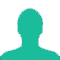# Half percent, written as a decimal, is

A. 0.2

B. 0.02

C. 0.005

D. 0.05

### Solution(By Examveda Team)

\eqalign{ & {\text{As}}\,{\text{we}}\,{\text{know}},\,1\% = \frac{1}{{100}} \cr & {\text{Hence}},\, \cr & \frac{1}{2}\% = {\frac{1}{2} \times \frac{1}{{100}}} \cr & \,\,\,\,\,\,\,\,\,\,\, = \frac{1}{{200}} \cr & \,\,\,\,\,\,\,\,\,\,\, = 0.005 \cr}

This Question Belongs to Arithmetic Ability >> Percentage

1.1/200 = 1/2 X 1/100 = 0.5 X 1/100 = 0.005

2.Very simple
O.5%
0.5/100
5/1000
0.005

3.How it is possible?

4.Hello, Sabet,
Can you explain your ans? How you get this? Thank You.

5.0.02

Related Questions on Percentage